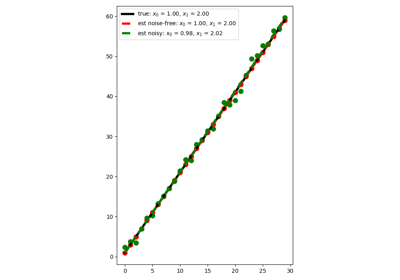# pylops.LinearRegression#

class pylops.LinearRegression(taxis, dtype='float64', name='L')[source]#

Linear regression.

Creates an operator that applies linear regression to a set of points. Values along the $$t$$-axis must be provided while initializing the operator. Intercept and gradient form the model vector to be provided in forward mode, while the values of the regression line curve shall be provided in adjoint mode.

Parameters
taxisnumpy.ndarray

Elements along the $$t$$-axis.

dtypestr, optional

Type of elements in input array.

Raises
TypeError

If taxis is not numpy.ndarray.

Regression

Polynomial regression

Notes

The LinearRegression operator solves the following problem:

$y_i = x_0 + x_1 t_i \qquad \forall i=0,1,\ldots,N-1$

We can express this problem in a matrix form

$\mathbf{y}= \mathbf{A} \mathbf{x}$

where

$\mathbf{y}= [y_0, y_1,\ldots,y_{N-1}]^T, \qquad \mathbf{x}= [x_0, x_1]^T$

and

$\begin{split}\mathbf{A} = \begin{bmatrix} 1 & t_{0} \\ 1 & t_{1} \\ \vdots & \vdots \\ 1 & t_{N-1} \end{bmatrix}\end{split}$

Note that this is a particular case of the pylops.Regression operator and it is in fact just a lazy call of that operator with order=1.

Attributes
shapetuple

Operator shape

explicitbool

Operator contains a matrix that can be solved explicitly (True) or not (False)

Methods

 __init__(taxis[, dtype, name]) adjoint() apply(t, x) Return values along y-axis given certain t location(s) along t-axis and regression coefficients x apply_columns(cols) Apply subset of columns of operator cond([uselobpcg]) Condition number of linear operator. conj() Complex conjugate operator div(y[, niter, densesolver]) Solve the linear problem $$\mathbf{y}=\mathbf{A}\mathbf{x}$$. dot(x) Matrix-matrix or matrix-vector multiplication. eigs([neigs, symmetric, niter, uselobpcg]) Most significant eigenvalues of linear operator. matmat(X) Matrix-matrix multiplication. matvec(x) Matrix-vector multiplication. reset_count() Reset counters rmatmat(X) Matrix-matrix multiplication. rmatvec(x) Adjoint matrix-vector multiplication. todense([backend]) Return dense matrix. toimag([forw, adj]) Imag operator toreal([forw, adj]) Real operator tosparse() Return sparse matrix. trace([neval, method, backend]) Trace of linear operator. transpose()

## Examples using pylops.LinearRegression#Linear Regression

Linear Regression